Case Study: Characterizing a TI DC/DC Converter

By Björn Rosqvist

Background/Problem

In IoT devices, it is vital to have an efficient power management system to get as much energy as possible out from the battery. One important part of this is to design an efficient DC/DC converter, boosting the voltage from the battery to the consumer. In this example, we use a 1.5 V alkaline battery to get a 3.3 V output. To get a high efficiency design, a lot of knowledge and measurements are required. A small IoT company usually has limited access to expensive measurement equipment so this article describes two cheap and quick design helps.

1. Help to calculate an efficiency value for a target system over the entire battery life, aiding the designer to choose the most efficient DC/DC converter and inductor.
2. Help to fully characterize one or several DC/DCs with different inductors, over its entire working range by using two Otii. In the end, the designer then can choose the best combination for best battery performance.

Measurement setup

Case 1

The Otii-Arc-001 from Qoitech AB (referred to as Otii in the rest of the article) acts as the battery, sweeping voltage from 1.5 V down to 0.9 V, measuring the efficiency by dividing the output energy from the DC/DC (Otii expansion port ADC measurement current and voltage) with the input energy to the DC/DC (Otii main current and voltage). The load is the DUT (device under test, i.e. target system). It is important to note, as will be discussed later, that the measurements should be long enough to ensure that the correct average is calculated.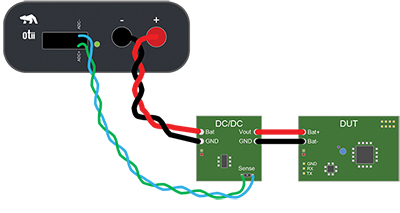Figure 1: Measurement setup for Case 1. (Image source: Qoitech AB)

For the setup shown in Figure 1, the DUT measures temperature, humidity, and light every 30 seconds and 10 such cycles are used for averaging. The total efficiency value is calculated by weighting how long the battery will remain at a given voltage level, see Figure 2, where it is estimated that the battery will be at 1.5 V for 9% of the time, 1.4 V for 8% of the time, etc. This is not completely correct but is an adequate estimation for this case.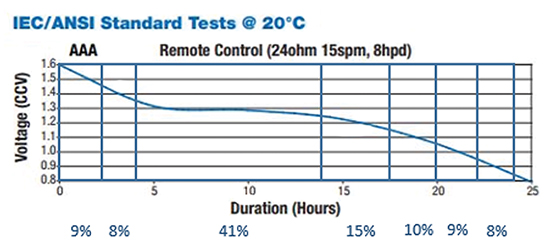Figure 2: AAA battery discharge profile. (Image source: Qoitech AB)

Case 2

One sourcing Otii acts as the battery, sweeping voltage from 1.5 V down to 0.9 V. This sourcing Otii is the one doing the measurements. The other Otii acts as a programmable constant current load, starting from 1 mA, 3 mA, 5 mA, 10 mA, 30 mA, 50 mA, and finally 90 mA (DC/DC upper limit is 100 mA).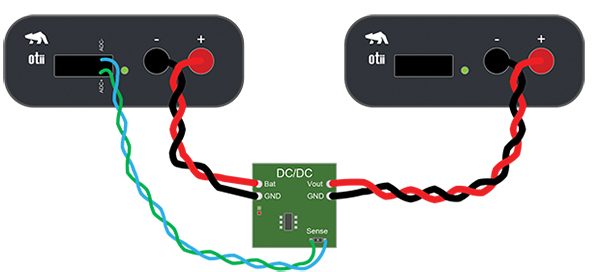Figure 3: Measurement setup for Case 2. (Image source: Qoitech AB)

The sourcing Otii measures efficiency by dividing the output energy (Otii expansion port ADC measurement current and voltage) with the input energy (Otii main current and voltage). Usually, the output voltage times the output current is divided with the input voltage times the input current, but since Otii calculates and displays the energy, it is much simpler to use this.

The Otii tool also enables the possibility to measure input and output voltage with four terminal sensing by using the SENSE+ and SENSE- inputs. This will not be discussed here due to the fairly low current and that Otii is connected to with short, low resistance cables.

Both Otiis, or as many Otiis that are connected, and all measurements (main current, main voltage, expansion port ADC current, expansion port ADC voltage, SENSE+, SENSE-, etc.) are presented in the same window, so it is very easy to show the data that is generated.

Results

Three different Texas Instruments DC/DCs were used in these cases.

As stated earlier, the measurements were taken over 10 periods for the DUT, that is 10 x 30 s = 5 min for each battery voltage. Figure 4 shows a screen capture of the TPS91097A-33DVBT DC/DC.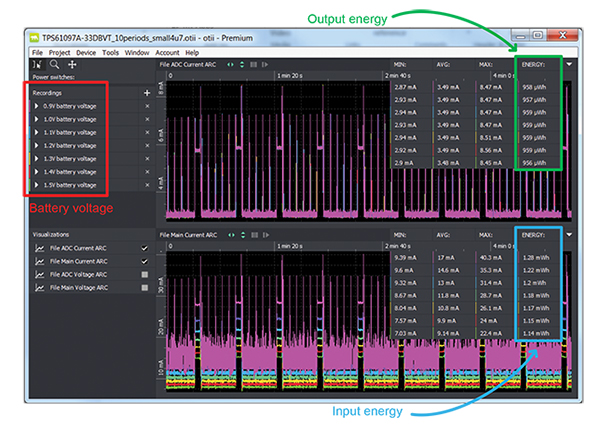Figure 4: Case 1 Otii measurement, TPS91097A-33DVBT. (Image source: Qoitech AB)

The Otii tool makes it very easy to calculate the efficiency by dividing the output energy by the input energy. That efficiency value is then weighted according to the description in measurement setup for Case 1. An overview of all three of the DC/DCs is shown in Figure 5.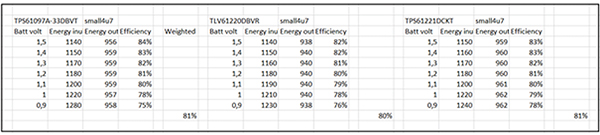Figure 5: Efficiency calculation for the different DC/DCs. (Image source: Qoitech AB)

This calculation can also be done automatically in the Otii with lua scripts (https://www.lua.org), but Figure 5 shows it in Excel to make it more visible.

The three DC/DCs performed almost the same with the small 4.7 µH chip inductor. To continue the DC/DC investigation, different inductors were used to see if there is an increase in the efficiency. Three different Bourns inductors and one Murata inductor were chosen.

The 22 µH inductor was way too big for this application, but it was interesting to see the performance.

The same setup as before was used with the TPS61097A-33DBVT chosen as the DC/DC and the inductor as the variable (Figure 6).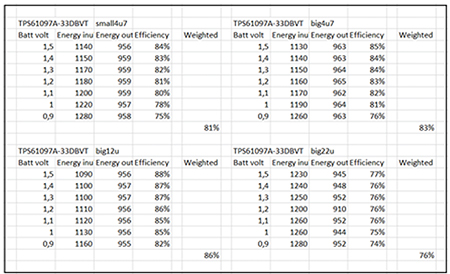Figure 6: Efficiency calculation for different inductors. (Image source: Qoitech AB)

The results were as expected, a higher efficiency DC/DC solution results with a bigger and lower resistance inductor. However, the huge 22 µH inductor is not the way to go.

To learn more about the behavior of the DC/DC, the case 2 was used in order to acquire a more in-depth characterization of the DC/DC over a range of input voltages and loads.

To start, Figure 7 looks at the measurements for the big 22 µH inductor. Figure 8 shows the same analysis on the other inductors.

Figure 7: Case 2, Otii measurement for TPS61097A-33DVBT with big 22 µH inductor. (Image source: Qoitech AB)

The sinking Otii starts with sinking 1 mA, then 3 mA, 5 mA, 10 mA, 30 mA, 50 mA, and finally 90 mA. This is repeated for all battery voltages.

As can be seen in the Figure 7, the DC/DC cannot handle 90 mA for the lower input voltages. The DC/DC can’t regulate for these low voltages and starts to oscillate.

The data was stored in .csv files that was imported by Matlab for easy analysis and plots. The efficiency is plotted in relation to the output current in Figure 8.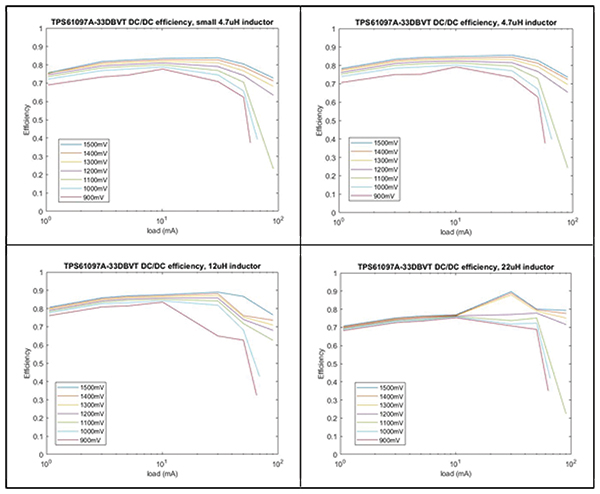Figure 8: Matlab graphs showing DC/DC efficiency for different inductors. (Image source: Qoitech AB)

This is a very good way to see the behavior of the DC/DC in different load conditions.

Conclusion

Otii is a very useful tool for easy analysis of a DC/DC’s efficiency, both in an intended system and for complete characterizations.

The three TI DC/DCs performed very similar in the simple system used in this analysis and the reason for choosing the TPS61097A-33DBVT was simply that it has a SOT23-5 package. Regarding the inductor choice, the 12 µH inductor should be chosen due to its higher efficiency and that there is space for it.